NEET  >  Assertion & Reason Test: Biological Classification

# Assertion & Reason Test: Biological Classification - NEET

Test Description

## 10 Questions MCQ Test - Assertion & Reason Test: Biological Classification

Assertion & Reason Test: Biological Classification for NEET 2023 is part of NEET preparation. The Assertion & Reason Test: Biological Classification questions and answers have been prepared according to the NEET exam syllabus.The Assertion & Reason Test: Biological Classification MCQs are made for NEET 2023 Exam. Find important definitions, questions, notes, meanings, examples, exercises, MCQs and online tests for Assertion & Reason Test: Biological Classification below.
Solutions of Assertion & Reason Test: Biological Classification questions in English are available as part of our course for NEET & Assertion & Reason Test: Biological Classification solutions in Hindi for NEET course. Download more important topics, notes, lectures and mock test series for NEET Exam by signing up for free. Attempt Assertion & Reason Test: Biological Classification | 10 questions in 20 minutes | Mock test for NEET preparation | Free important questions MCQ to study for NEET Exam | Download free PDF with solutions
 1 Crore+ students have signed up on EduRev. Have you?
Assertion & Reason Test: Biological Classification - Question 1

### Directions : Each of these questions contain two statements, Assertion and Reason. Each of these questions also has four alternative choices, only one of which is the correct answer. You have to select one of the codes (a), (b), (c) and (d) given below.Assertion : If dot product and cross product of A and B are zero, it implies that one of the vector A and B must be a null vectorReason : Null vector is a vector with zero magnitude.

Detailed Solution for Assertion & Reason Test: Biological Classification - Question 1
If →A and →B are not null Vectors then it follows that sinθ and cosθ both should be zero simultaneously. But it cannot be possible so it is essential that one of the vectors must be a null vector.
Assertion & Reason Test: Biological Classification - Question 2

### Directions : Each of these questions contain two statements, Assertion and Reason. Each of these questions also has four alternative choices, only one of which is the correct answer. You have to select one of the codes (a), (b), (c) and (d) given below.Assertion: A physical quantity cannot be called as a vector if its magnitude is zero.Reason: A vector has both magnitude and direction.

Detailed Solution for Assertion & Reason Test: Biological Classification - Question 2
If a vector quantity has zero magnitude then it is called a null vector. That quantity may have some direction even if its magnitude is zero.
Assertion & Reason Test: Biological Classification - Question 3

### Directions : Each of these questions contain two statements, Assertion and Reason. Each of these questions also has four alternative choices, only one of which is the correct answer. You have to select one of the codes (a), (b), (c) and (d) given below.Assertion : If A.B= B.C, then A may not always be equal to C.Reason : The dot product of two vectors involves cosine of the angle between the two vectors.

Detailed Solution for Assertion & Reason Test: Biological Classification - Question 3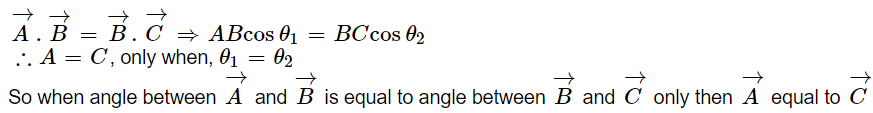Assertion & Reason Test: Biological Classification - Question 4

Directions : Each of these questions contain two statements, Assertion and Reason. Each of these questions also has four alternative choices, only one of which is the correct answer. You have to select one of the codes (a), (b), (c) and (d) given below.

Assertion : The magnitude of velocity of two boats relative to river is same. Both boats start simultaneously from same point on one bank may reach opposite bank simultaneously moving along different paths.

Reason : For boats to cross the river in same time. The component of their velocity relative to river in direction normal to flow should be same.

Detailed Solution for Assertion & Reason Test: Biological Classification - Question 4
If component of velocity of boat relative to river is same normal to river flow (as shown in figure) both boats reach other bank simultaneously since this component is responsible for reaching the other bank of the river.
Assertion & Reason Test: Biological Classification - Question 5

Directions : Each of these questions contain two statements, Assertion and Reason. Each of these questions also has four alternative choices, only one of which is the correct answer. You have to select one of the codes (a), (b), (c) and (d) given below.

Assertion : τ=r x F and τ=F x r

Reason : Cross product of vectors is commutative.

Detailed Solution for Assertion & Reason Test: Biological Classification - Question 5
Cross-product of two vectors is anticommutative.

Cross product of vectors is not commutative.

Assertion & Reason Test: Biological Classification - Question 6

Directions : Each of these questions contain two statements, Assertion and Reason. Each of these questions also has four alternative choices, only one of which is the correct answer. You have to select one of the codes (a), (b), (c) and (d) given below.

Assertion : If there were no gravitational force, the path of the projected body always be a straight line.

Reason : Gravitational force makes the path of projected body always parabolic.

Detailed Solution for Assertion & Reason Test: Biological Classification - Question 6
Upto ordinary heights, the gravitational force remains stationary, since the distance from earth's surface is negligible compared to radius of the earth. Thus the path of projectiles is parabolic.

However as the distance increases, the gravitational field decreases inversely as square of distance from earth. Thus the gravitational field is variable. Under such a gravitational field, the path of projectile is elliptical.

Assertion & Reason Test: Biological Classification - Question 7

Directions : Each of these questions contain two statements, Assertion and Reason. Each of these questions also has four alternative choices, only one of which is the correct answer. You have to select one of the codes (a), (b), (c) and (d) given below.

Assertion : Two balls of different masses are thrown vertically upward with same speed. They will pass through their point of projection in the downward direction with the same speed.

Reason : The maximum height and downward velocity attained at the point of projection are independent of the mass of the ball.

Detailed Solution for Assertion & Reason Test: Biological Classification - Question 7
Let the balls be thrown vertically upward with speed u.

Using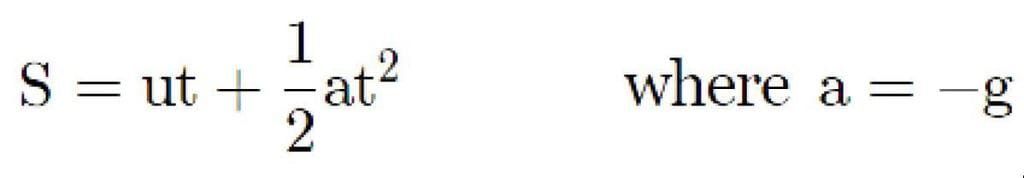Therefore, the Maximum height,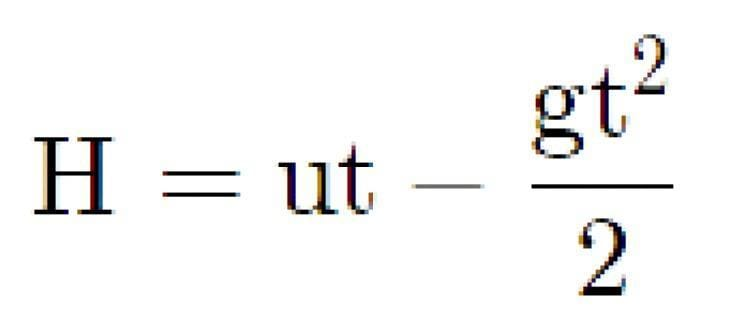Also using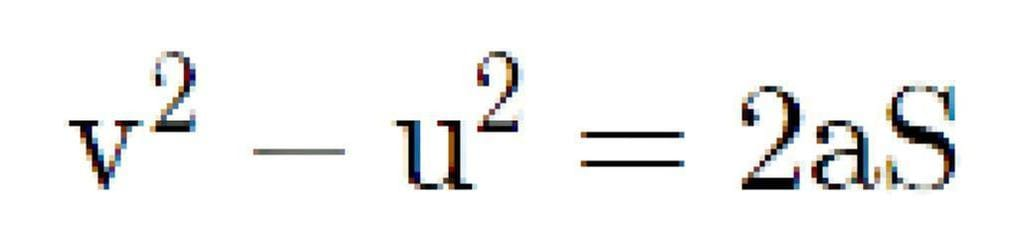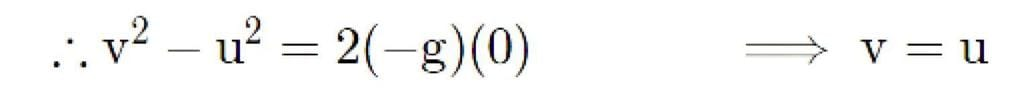Thus the balls will pass through the point of projection in downward direction with the same speed (v). Also from the equations used, the maximum height and the downward velocity attained are independent of mass of the ball.

Assertion & Reason Test: Biological Classification - Question 8

Directions : Each of these questions contain two statements, Assertion and Reason. Each of these questions also has four alternative choices, only one of which is the correct answer. You have to select one of the codes (a), (b), (c) and (d) given below.

Assertion : If a body of mass m is projected upwards with a speed V making an angle ? with the vertical, than the change in the momentum of the body along X–axis is zero.

Reason : Mass of the body remains constant along X–axis

Detailed Solution for Assertion & Reason Test: Biological Classification - Question 8
When a body is projected up making an angle ? the velocity component along-axis remains constant. Therefore, Momentum along x-axis is constant. Along horizontal, mass and velocity both are constant.
Assertion & Reason Test: Biological Classification - Question 9

Directions : Each of these questions contain two statements, Assertion and Reason. Each of these questions also has four alternative choices, only one of which is the correct answer. You have to select one of the codes (a), (b), (c) and (d) given below.

Assertion : Minimum number of non-equal vectors in a plane required to give zero resultant is three.

Reason : If sum of vectors A+B+C=0 , then they must lie in one plane.

Detailed Solution for Assertion & Reason Test: Biological Classification - Question 9
As the vectors are non-equal so two vector can not make zero resultant. According to triangle law of vector addition at least 3 vectors are needed to make zero resultant. Thus, we can say that statement -2 is the reason of statement -1.
Assertion & Reason Test: Biological Classification - Question 10

Directions : Each of these questions contain two statements, Assertion and Reason. Each of these questions also has four alternative choices, only one of which is the correct answer. You have to select one of the codes (a), (b), (c) and (d) given below.

Assertion : The scalar product of two vectors can be zero.

Reason : If two vectors are perpendicular to each other, their scalar product will be zero.

Detailed Solution for Assertion & Reason Test: Biological Classification - Question 10
If θ be the angle between two vectors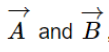, then their scalar product,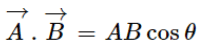If θ = 90 degrees then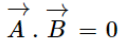I.e. ifare perpendicular to each other then their scalar product will be zero.

Information about Assertion & Reason Test: Biological Classification Page
In this test you can find the Exam questions for Assertion & Reason Test: Biological Classification solved & explained in the simplest way possible. Besides giving Questions and answers for Assertion & Reason Test: Biological Classification, EduRev gives you an ample number of Online tests for practice

### How to Prepare for NEET

Read our guide to prepare for NEET which is created by Toppers & the best Teachers(Scan QR code)